Download Presentation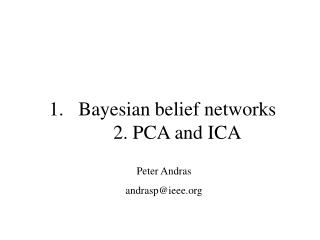Bayesian belief networks 2. PCA and ICA

# Bayesian belief networks 2. PCA and ICA - PowerPoint PPT Presentation

Bayesian belief networks 2. PCA and ICA. Peter Andras andrasp@ieee.org. Principal component analysis PCA 1. Idea: the high dimensional data might be situated on a lower dimensional surface. PCA 2. How to find the lower dimensional surface ?. We look for linear surfaces, i.e., hyperplanes.I am the owner, or an agent authorized to act on behalf of the owner, of the copyrighted work described.
Download Presentation## Bayesian belief networks 2. PCA and ICA

An Image/Link below is provided (as is) to download presentation

Download Policy: Content on the Website is provided to you AS IS for your information and personal use and may not be sold / licensed / shared on other websites without getting consent from its author.While downloading, if for some reason you are not able to download a presentation, the publisher may have deleted the file from their server.

- - - - - - - - - - - - - - - - - - - - - - - - - - E N D - - - - - - - - - - - - - - - - - - - - - - - - - -
Presentation Transcript### Bayesian belief networks2. PCA and ICA

Peter Andras

andrasp@ieee.org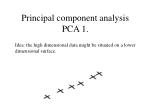Principal component analysisPCA 1.

Idea: the high dimensional data might be situated on a lower dimensional surface.PCA 2.

How to find the lower dimensional surface ?

We look for linear surfaces, i.e., hyperplanes.

We decompose the correlation matrix of data conform its eigenvectors.PCA 3.

The eigenvectors are called principal component vectors.

The new data vectors are formed by the projections of the original data vectors onto the principal component vectors.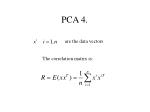PCA 4.

are the data vectors

The correlation matrix is: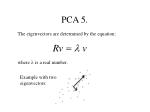PCA 5.

The eigenvectors are determined by the equation:

where  is a real number.

Example with two eigenvectors: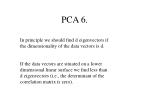PCA 6.

In principle we should find d eigenvectors if the dimensionality of the data vectors is d.

If the data vectors are situated on a lower dimensional linear surface we find less than d eigenvectors (i.e., the determinant of the correlation matrix is zero).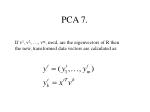PCA 7.

If v1, v2, …, vm, m<d, are the eigenvectors of R then the new, transformed data vectors are calculated as: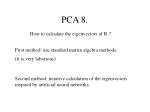PCA 8.

How to calculate the eigenvectors of R ?

First method: use standard matrix algebra methods.

(it is very laborious)

Second method: iterative calculation of the eigenvectors inspired by artificial neural networks.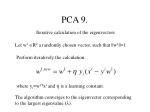PCA 9.

Iterative calculation of the eigenvectors

Let w1 Rd a randomly chosen vector, such that ||w1||=1

Perform iteratively the calculation:

where yi=w1Txi and  is a learning constant.

The algorithm converges to the eigenvector corresponding to the largest eigenvalue ().PCA 10.

To calculate the following eigenvectors we modify the iterative algorithm. Now we use the calculation formula:

where

and uji=wjTxi.

This iterative algorithm converges to wk the k-th eigenvector.PCA 11.

If the algorithm doesn’t converge the situation can be:

a. the vector enters in a cycle;

b. the values doesn’t form any cycle.

If we have a cycle, all the vectors of the cycle are eigenvectors, and their corresponding eigenvalues are very close.

If we have no convergence and no cycle, that means that there is no more eigenvector that can be determined.PCA 12.

How to use the PCA for dimension reduction ?

Select the important eigenvectors.

Many times all of the eigenvectors can be determined but only part of them are important.

The importance of the eigenvectors is shown by their associated eigenvalue.PCA 13.

Selecting the important eigenvectors.

1. Graphical method:PCA 14.

Selecting the important eigenvectors.

2. Relative power:

3. Cumulative power:PCA 15.

Summary

The PCA is used for dimensionality reduction.

The data vectors are projected on the eigenvectors of their correlation matrix to obtain the transformed data vectors.

To calculate easily the PCA we can use the iterative algorithm.

To reduce the data dimension we consider only the important eigenvectors.Independent component analysisICA 1.

The idea: if the data vectors are linear combination of statistically independent data components, they should be separable in their components.

This is true if the component vectors have non-Gaussian distribution, with sharper or flatter peak.ICA 2.

Suppose xi=Asi, where xi are the data vectors, si are the vectors of statistically independent components (sji)

Our goal is to find the matrix A (more precisely, the rows of it).

Example: ‘cocktail-party’ effect: many independent voices registered together; goal: separate the independent voices; the recorded mixture is a linear mixture.ICA 3.

How to find the independent components ?

Optimize:

All solution vectors (w) are local minimum solutions, and they correspond to one of the independent components, i.e., on the components of the si vectors.ICA 4.

How to do it practically ?

FastICA algorithm (Hyvarinen and Oja):

Calculates by iterations the w vectors.

The calculation formula is:

w converges to one of the vectors corresponding to one of the independent components.ICA 5.

In practice we have to calculate several w vectors. To test whether the generated independent components are really independent we can use statistical tests.

Let us consider s1i=w1Txi and s2i=w2Txi.

Then we can test the independence of s1 and s2 by calculating their correlation and testing their identical origin by the F-test (they may not be strongly correlated but at the same time they may have identical origin).

If the testing accepts the independence of the two series we may accept w2 as a new vector that corresponds to a separate independent component.ICA 6.

Remarks

By calculating the independent components we get a new representation of the data, which has the property that the components contain minimum mutual information.

We can use the ICA to select the independent non-Gaussian components, but we cannot separate the Gaussian mixtures.